Computational & Technology Resources
an online resource for computational,
engineering & technology publications
Civil-Comp Proceedings
ISSN 1759-3433
CCP: 84
PROCEEDINGS OF THE FIFTH INTERNATIONAL CONFERENCE ON ENGINEERING COMPUTATIONAL TECHNOLOGY
Edited by: B.H.V. Topping, G. Montero and R. Montenegro
Paper 216

Sequential Numerical Study of some Variations of a Second-Degree Iterative Method: Application to the Neutron Diffusion Equation

O. Flores12, V. Vidal2, G. Verdú3, J. Garayoa2 and P. Flores4

1Department of Computer Science, Tuxtepec Institute of Technology, Oaxaca, Mexico
2Department of Information Systems and Computing, 3Department of Chemical and Nuclear Engineering, Polytechnic University of Valencia, Spain
4Technical School of Electrical Engineering "El Recreo", Veracruz, Mexico

Full Bibliographic Reference for this paper
O. Flores, V. Vidal, G. Verdú, J. Garayoa, P. Flores, "Sequential Numerical Study of some Variations of a Second-Degree Iterative Method: Application to the Neutron Diffusion Equation", in B.H.V. Topping, G. Montero, R. Montenegro, (Editors), "Proceedings of the Fifth International Conference on Engineering Computational Technology", Civil-Comp Press, Stirlingshire, UK, Paper 216, 2006. doi:10.4203/ccp.84.216
Keywords: second-degree iterative methods, neutron diffusion equation, large-sparse linear systems, Krylov subspace methods, transient analysis, energy groups.

Summary
For design and safety reasons, nuclear power plants need fast and accurate plant simulators. The centre point of concern in the simulation of a nuclear power plant is the reactor core. Since it is the source of the energy that is produced in the reactor, a very accurate model of its processes is needed. The neutron population into the reactor core is modeled using the Boltzmann transport equation. This three-dimensional problem is modeled by a complicated system of coupled partial differential equations, the multigroup neutron diffusion equation[1,2], that have been discretised using a nodal collocation method in space and one-step backward-difference method in time. The solution of these equations can involve very intensive computing. Therefore, it is necessary to find effective algorithms for the solution of the three-dimensional model.

Bru et al.  applied two Second-Degree methods (A and B methods)  to solve the linear system of equations related to a two-dimensional neutron-diffusion equation case. In our work, we test these methods to solve the linear system of equations related to a three-dimensional neutron-diffusion equation for the case of the commercial reactor of Leibstadt .

Thus, when the neutron-diffusion equation is discretised in space and time, it is necessary to solve a large and sparse system of linear equations with the following block structure for each time step: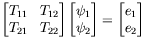(116)

In addition to the A and B methods to solve (116), we propose the following operations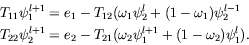(117)

In scheme (117), named method C, we have added two different parameters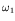and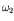for each system to be computed, in order to accelerate its convergence.

Besides to method C, we have carried out experiments with an `adaptable' technique, achieving some improvements in the process efficiency. This technique computes the solution of the systems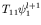and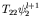with a cheap precision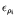at initial stages of the method. Then, this precision is 'adapted' or 'improved' towards a more demanding one in successive iterations.

The application of this technique to method C gives the following algorithm, which we have named method D in present work, and we have obtained an acceptable performance in comparison to the others methods presented in this work.

(1) Set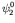;     {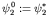}

(2) Set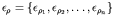where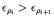;

(2) Solve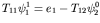(3) Solve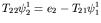(4) For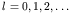(4a) Solve for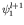with tolerance(4b) Solve for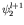with tolerance(4c) if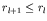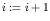end if

until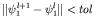and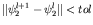References
1
J.R. Weston, M. Stacey, "Space-Time Nuclear Reactor Kinetics", Academic Press, 1970.
2
A.F. Henry, "Nuclear Reactor Analysis", The M.I.T Press, 1975.
3
R. Bru, D. Ginestar, J. Marín, G. Verdú, J. Mas, T. Manteuffel, "Iterative Schemes for the Neutron Diffusion Equation", Computers and Mathematics with Applications, 44, 1307-1323, 2002. doi:10.1016/S0898-1221(02)00258-4
4
D.M. Young, Iterative Solution of Large Linear Systems, Academic Press Inc., New York, 1971.
5
J. Blomstrand, The KKL Core Stability Test, conducted in September 1990, ABB Report, BR91-245, 1992.

purchase the full-text of this paper (price £20)